Logic Gates MCQ

# Logic Gates MCQ

Test Description

## 10 Questions MCQ Test Topic wise Tests for IIT JAM Physics | Logic Gates MCQ

Logic Gates MCQ for Physics 2023 is part of Topic wise Tests for IIT JAM Physics preparation. The Logic Gates MCQ questions and answers have been prepared according to the Physics exam syllabus.The Logic Gates MCQ MCQs are made for Physics 2023 Exam. Find important definitions, questions, notes, meanings, examples, exercises, MCQs and online tests for Logic Gates MCQ below.
Solutions of Logic Gates MCQ questions in English are available as part of our Topic wise Tests for IIT JAM Physics for Physics & Logic Gates MCQ solutions in Hindi for Topic wise Tests for IIT JAM Physics course. Download more important topics, notes, lectures and mock test series for Physics Exam by signing up for free. Attempt Logic Gates MCQ | 10 questions in 30 minutes | Mock test for Physics preparation | Free important questions MCQ to study Topic wise Tests for IIT JAM Physics for Physics Exam | Download free PDF with solutions
 1 Crore+ students have signed up on EduRev. Have you?
Logic Gates MCQ - Question 1

### The output of an AND gate with 3 inputs A, B and C is high when :

Detailed Solution for Logic Gates MCQ - Question 1

The correct answer is :A = 1 , B = 1 , C = 1

Logic Gates MCQ - Question 2

### How many input possibilities for a 3 input AND gate will result in the HIGH output?

Detailed Solution for Logic Gates MCQ - Question 2

Logic Gates MCQ - Question 3

### What is the expression for a 4 input OR gate?

Detailed Solution for Logic Gates MCQ - Question 3

The correct answer is: Y= A + B + C + D

Logic Gates MCQ - Question 4

If a signal passing through a gate is inhibited by sending a LOW into one of the inputs and the output is HIGH, the gate is a(n)

Detailed Solution for Logic Gates MCQ - Question 4

Logic Gates MCQ - Question 5

It is required to change a logic 1 to logic 0 and vice versa the logic gate required to do this is :

Detailed Solution for Logic Gates MCQ - Question 5

Logic Gates MCQ - Question 6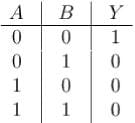What gate corresponds to the above logic gate?

Detailed Solution for Logic Gates MCQ - Question 6

The correct answer is: NOR gate

Logic Gates MCQ - Question 7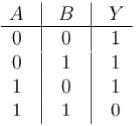What gate corresponds to the logic gate shown above :

Detailed Solution for Logic Gates MCQ - Question 7

Logic Gates MCQ - Question 8

Outputs from an AND gate and OR gate are fed into a 2 input NAND gate the output is :

Detailed Solution for Logic Gates MCQ - Question 8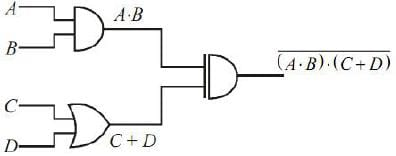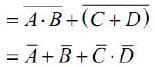The correct answer is: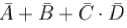Logic Gates MCQ - Question 9

Which of the following is true fo r an exclusive OR gate?

Detailed Solution for Logic Gates MCQ - Question 9

The correct answer is: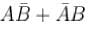Logic Gates MCQ - Question 10

If a 3 input NOR gate has eight input possibilities how many of them will result in a high output?

Detailed Solution for Logic Gates MCQ - Question 10

## Topic wise Tests for IIT JAM Physics

217 tests
 Use Code STAYHOME200 and get INR 200 additional OFF Use Coupon Code
Information about Logic Gates MCQ Page
In this test you can find the Exam questions for Logic Gates MCQ solved & explained in the simplest way possible. Besides giving Questions and answers for Logic Gates MCQ, EduRev gives you an ample number of Online tests for practice

217 tests